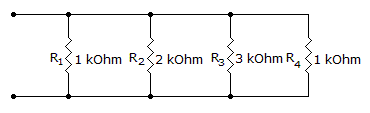# Electronics - Parallel Circuits - Discussion

Discussion Forum : Parallel Circuits - General Questions (Q.No. 23)
23.
What is the total resistance?7 k1 k706353Explanation:
No answer description is available. Let's discuss.
Discussion:
20 comments Page 1 of 2.

Anuja said:   3 years ago
I don't know total resistance in parallel (1/R1)+(1/R2)+(1/R3)+(1/R4).

Anuja said:   3 years ago
I don't understand total resistance in parallel (1/R1)+( 1/R2)+(1/R3)+(1/R4) so please give me answer and explain in simple words?

Ponman Peter said:   6 years ago
I'm not getting for the fact that only R2 is given and we're required to calculate total resistance.

1/1000+1/2000+1/3000+1/1000=0.00283.

1/0.00283=353.

Abhi said:   7 years ago
Try to send current through one of the points from open circuit side and try to reduce it by parallel rule.

Krushna waghmare said:   9 years ago
RT = 1/R1+1/R2+1/R3+1/R4........1/RN formula 1/1000, 1/2000, 1/3000, 1/1000.

RT = 5+2.5+1.66+5/5000 Lasavi.

= 14.16/5000 Moho.

= 5000/14.16 ohm.

Aastha said:   9 years ago
Sorry @Ragavignesham I am unable to understand. Can you please explain in more words?

Hirenkumar said:   10 years ago
It is very simple ,
If we calulate like this,
Here,
R1=1k.
R2=2k.
R3=3k.
R4=1k.

If we do R1||R4 then simply 1k||1k=500 Ohms.
So final answer should be less than 500 ohms as there are R2 and R3 are there.

And in option there is only one option which is less than 500 Ohms.

Hello Friends.

There are three resistance values...say R1, R2, R3, R4.

First calculate R1||R2 = 2/3 = r1 then, r1||R3 = 6/11 = r2 then, r2||R4 = 0.3529K = 353 ohm.

Finally R1||R2||R3||R4 = 353 ohm.

Ali Umar said:   1 decade ago
1/Requ = 1/R1 + 1/R2 + 1/R3 = 1/1 + 1/2 + 1/3 + 1/1 = 17/6 kohm.

Requ = 6/17 Kohm.

Now convert 6/17 kohm into ohm so multiply with 1000(1kohm=1000ohm).

So 6/17*1000 = 352.94.